# 12-267/Homework Assignment 8

This assignment is due in class on Tuesday November 27. Here and everywhere, neatness counts!! You may be brilliant and you may mean just the right things, but if your readers have a hard time deciphering your work they will give up and assume it is wrong.

Task 1. Find the singular (that is, non-ordinary) points of the equation below, and for each one decide if it is regular or not: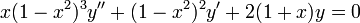$x(1-x^2)^3y''+(1-x^2)^2y'+2(1+x)y=0$

Task 2. Find the general solution of the following two equations:

1.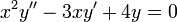$x^2y''-3xy'+4y=0$
2.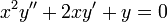$x^2y''+2xy'+y=0$

(You are allowed to use complex numbers within the derivation, but your solutions should be real-valued).

Task 3. Using power series, find two linearly independent solutions for each of the equations

1.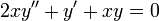$2xy''+y'+xy=0$
2.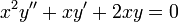$x^2y''+xy'+2xy=0$

Task 4. An equation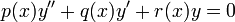$p(x)y''+q(x)y'+r(x)y=0$ is said to have a regular singular at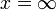$x=\infty$ if the equation obtained from it by the change of substitution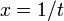$x=1/t$ has a regular singular point at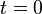$t=0$. Write explicitly the conditions on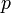$p$,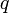$q$, and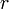$r$ that this entails.

 Dror's notes above / Student's notes below

Solutions to HW8: Dongwoo.kang